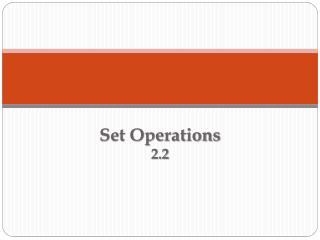DownloadDownload PresentationSet Operations 2.2

# Set Operations 2.2

Download Presentation## Set Operations 2.2

- - - - - - - - - - - - - - - - - - - - - - - - - - - E N D - - - - - - - - - - - - - - - - - - - - - - - - - - -
##### Presentation Transcript

1. Set Operations2.2

2. Intersection of Sets The intersection of sets A and B, denoted by , is the set of all elements that are common to both. That is, . Union of Sets The union of sets A and B, denoted by , is the set of all elements that are either in A or B or in both. That is, .

3. Let A = {1,2,3,4,5}, B = {1,3,4,6}, and C = {1,6,7}. Find the following: B  C A  B A (B C) SOLUTION 1. B  C = {1, 6} 2. A  B = {1, 2, 3, 4, 5, 6} 3. A (B C) = {1, 2, 3, 4, 5, 6}

4. Complement of a Set Let Ube the universal set, and let A be a subset of U . The complement of A, denoted by A, is the set of elements in U that are not in A. That is, A = {x | x Uandx A}. This set is also symbolized by U – A . De Morgan’s Law For any sets A and B, (AB)’ = A’ B’ (AB)’ = A’  B’

5. Let U = {a, b, c, d, e, f, g}, A = {a, c, e}, B = {b, c, e, f, g}, C = {a, c, d, f} and D = {a, d, g}. Find each specified set. • (A  B)’ • (A  B)’ • (A  C)  B’ • (C’  A)  D SOLUTION 1. (A  B) = {c, e} 2. (A  B) = {a, b, c, d, e, f, g} (A  B)’ = {a, b, d, f, g} (A  B)’ = Ø 3. (A  C) = {a, c, d, e, f} and B’ = {a, d} (A  C)  B’ = {a, d} 4. C’ = {b, e, g} and A = {a, c, e} (C’  A) = {a, b, c, e, g} and D = {a, d, g} (C’  A)  D = {a, g}

6. Difference of Sets If A and B are two sets, the difference of A and B, denoted by A – B, is the set of all elements that are in A and not in B. That is, A  B = {x | x A andx B}. Let U = {1, 2, 3, 4, 5}, A = {2, 3, 4}, and B = {1, 4, 5}. Find each specified set. a. U B b . B  A c. B a.U B = {2, 3} ANSWERS b . B  A = {1, 5} c. B = {2, 3}

7. SOLUTION a. U B b . B  A {1, 2, 3, 4, 5}  {1, 4, 5} {1, 4, 5}  {2, 3, 4} {1, 2, 3, 4, 5}  {1, 4, 5} {1, 4, 5}  {2, 3, 4} {2, 3} {1, 5} c. B {1, 2, 3, 4, 5} {2, 3}